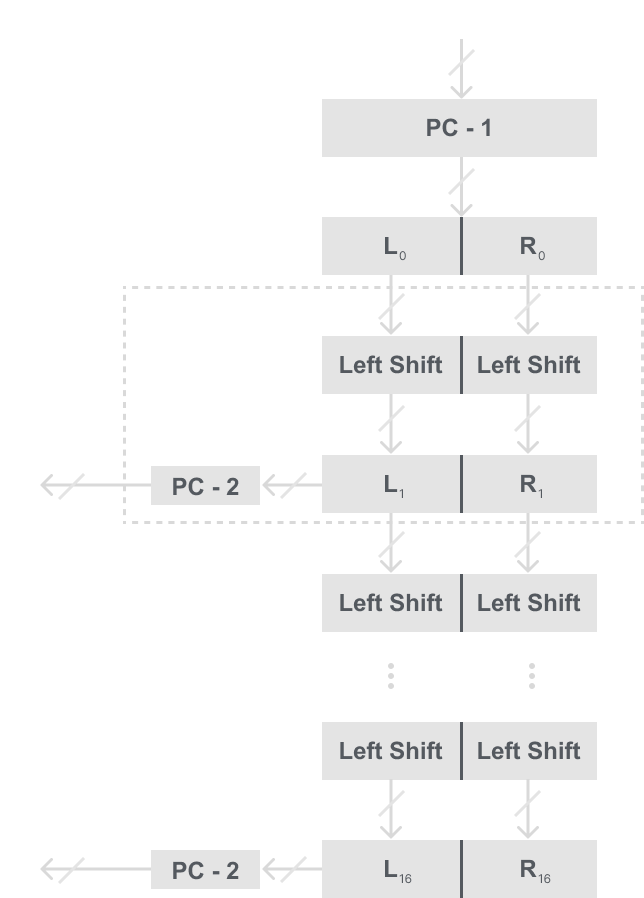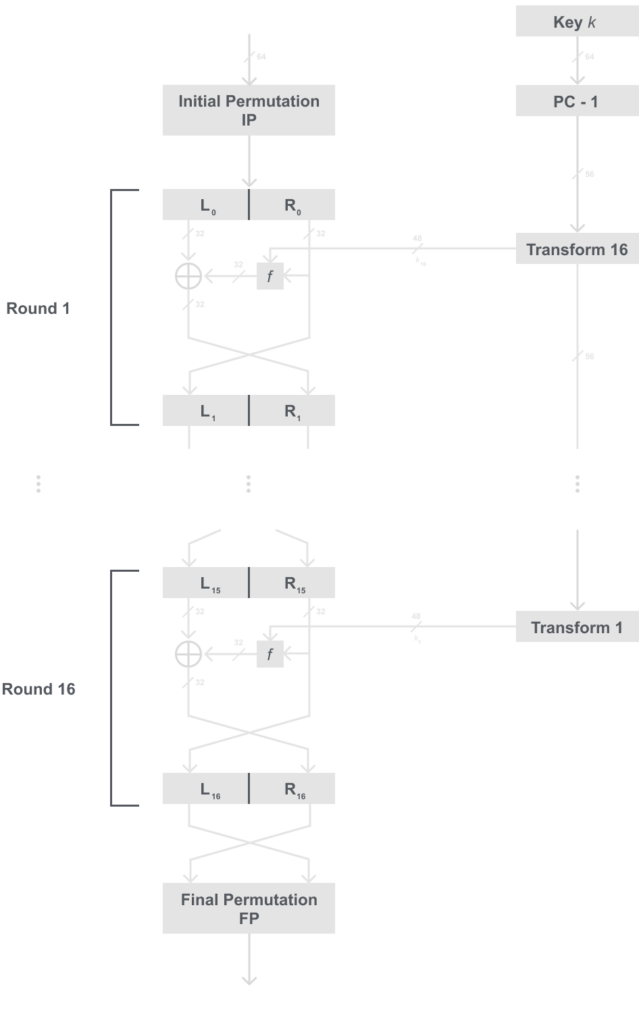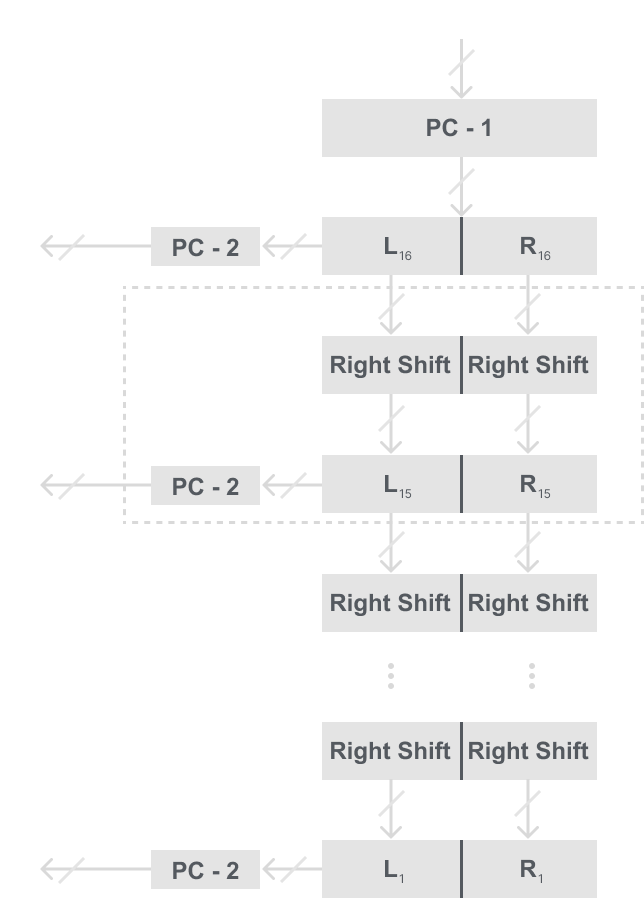Back To All

# (DES) Key Schedule and Decryption [Part 2]This is a continuation of (DES) The Data Encryption Standard [Part 1], so it is highly encouraged to read that post before reading this post. In that post I gave the history of DES and also explained the internals of the DES algorithm.

In this post we will discuss two things:

• The DES Key schedule
• Decryption of DES

## The Key Schedule of DES

The thing I glossed over in Part 1 was how we obtain the 16 round keys that were present in the f-function. First, let me give a description of the key schedule, then I will give the normal diagram and break it down further.

The key schedule derives 16 round keys ki, each consisting of 48 bits from the original 64-bit key. Note that I will also refer to these as subkeys, which is another term for round keys. You may be wondering why I stated that the original key was 64 bits, this is because every eighth bit is removed in the first permutation round. During research for this post, I could not find a definitive answer to why this is the case. All I can say for sure is that the 8 bits removed do not add to the actual security of DES, and the key space of DES is still 256.

As seen in the figure below, the 64-bit original key is reduced to 56-bits by ignoring every eight bit in the initial permutation PC-1 which stands for “Permuted Choice one”

### Initial key Permutation Table PC-1

 57 49 41 33 25 17 9 1 58 50 42 34 26 18 10 2 59 51 43 35 27 19 11 3 60 52 44 36 63 55 47 39 31 23 15 7 62 54 46 38 30 22 14 6 61 53 45 37 29 21 13 5 28 20 12 4

The resulting 56-bit key from PC-1 is split into two 28-bit halves L and R. Each half is then rotated 1 or 2 positions left according on what round it is based on these rules:

• rotate 1:    if i = 1, 2, 9, 16
• rotate 2:   if any other round

It is important to notice that the total number of rotations is: 4 ⋅ 1 + 12 ⋅ 2 = 28. This leads to the interesting fact that L0 = L16 and R0 = R16. This fact will be very useful in the decryption algorithm seen later.

### Key schedule diagramNotice from the model that to get our 48-bit round key we first have to combine our two shifted halves then put them into our second permutation PC-2 called “Permuted Choice two”

In PC-2 it takes a 56-bit input and gives a 48-bit output that we use as our round, the exact bitwise permutation PC-2 table is below:

### Second Permutation Table PC-2

 14 17 11 24 1 5 3 28 15 6 21 10 23 19 12 4 26 8 16 7 27 20 13 2 41 52 31 37 47 55 30 40 51 45 33 48 44 49 39 56 34 53 46 42 50 36 29 32

It is important to see that each round key is merely a permutation of the original 56-bit key, thus the key schedule was designed in such a way that each permutation is used in a different round.

## Decryption in DES

One advantage of DES is that it is a Feistel network. Therefore, decryption and encryption are essentially the same functions. When compared to encryption the only thing that changes is that the key schedule is reversed. So instead of starting with round k1 in encryption, we start with round key k16 decryption.

Here is a model of decryption in DES:Something you may notice, is first it may be hard to tell a difference in the encryption and decryption model, that is by design. The only thing that changed was the order of the key schedule the rest of the algorithm is the exact same.

Therefore, we just have to change our key schedule in order to be able to inverse it. The only thing we actually need to change is how we shift the key. Thus, instead of shifting it left, we shift it right. We will show the changes in the diagram below:Recall that I said before that L0 = L16 and R0 = R16. This is the fact that allows us to reuse the DES algorithm for both encryption and decryption.

For this reason, DES is still a very popular cipher because it is relatively small in hardware.

## Code Implementation

Here is my code implementation of DES in C++ this is done for educational purposes, and most ciphers are best suited for hardware. This implementation for my blog will only have the key schedule and encryption for simplicity. If you want to see a more organized program with decryption make sure to check out my GitHub here.

```				```
#include <iostream>
#include <cmath>
#include <string>

const int PC1 = { 57, 49, 41, 33, 25, 17, 9, 1,
58, 50, 42, 34, 26, 18, 10, 2,
59, 51, 43, 35, 27, 19, 11, 3,
60, 52, 44, 36, 63, 55, 47, 39,
31, 23, 15, 7, 62, 54, 46, 38,
30, 22, 14, 6, 61, 53, 45, 37,
29, 21, 13, 5, 28, 20, 12, 4 };

const int PC2 = { 14, 17, 11, 24, 1, 5, 3, 28,
15, 6, 21, 10, 23, 19, 12, 4,
26, 8, 16, 7, 27, 20, 13, 2,
41, 52, 31, 37, 47, 55, 30, 40,
51, 45, 33, 48, 44, 49, 39, 56,
34, 53, 46, 42, 50, 36, 29, 32 };

const int IP = { 58, 50, 42, 34, 26, 18, 10, 2,
60, 52, 44, 36, 28, 20, 12, 4,
62, 54, 46, 38, 30, 22, 14, 6,
64, 56, 48, 40, 32, 24, 16, 8,
57, 49, 41, 33, 25, 17, 9, 1,
59, 51, 43, 35, 27, 19, 11, 3,
61, 53, 45, 37, 29, 21, 13, 5,
63, 55, 47, 39, 31, 23, 15, 7 };

int FP = { 40, 8, 48, 16, 56, 24, 64, 32,
39, 7, 47, 15, 55, 23, 63, 31,
38, 6, 46, 14, 54, 22, 62, 30,
37, 5, 45, 13, 53, 21, 61, 29,
36, 4, 44, 12, 52, 20, 60, 28,
35, 3, 43, 11, 51, 19, 59, 27,
34, 2, 42, 10, 50, 18, 58, 26,
33, 1, 41, 9, 49, 17, 57, 25 };

const int E = { 32, 1, 2, 3, 4, 5,
4 ,5, 6, 7, 8, 9,
8, 9, 10, 11, 12, 13,
12, 13, 14, 15, 16, 17,
16, 17, 18, 19, 20, 21,
20, 21, 22, 23, 24, 25,
24, 25, 26, 27, 28, 29,
28, 29, 30, 31, 32, 1 };

const int P = { 16, 7, 20, 21, 29, 12, 28, 17,
1, 15, 23, 26, 5, 18, 31, 10,
2, 8, 24, 14, 32, 27, 3, 9,
19, 13, 30, 6, 22, 11, 4, 25 };

const int substition_boxes ={ { 14,4,13,1,2,15,11,8,3,10,6,12,5,9,0,7,
0,15,7,4,14,2,13,1,10,6,12,11,9,5,3,8,
4,1,14,8,13,6,2,11,15,12,9,7,3,10,5,0,
15,12,8,2,4,9,1,7,5,11,3,14,10,0,6,13 },

{ 15,1,8,14,6,11,3,4,9,7,2,13,12,0,5,10,
3,13,4,7,15,2,8,14,12,0,1,10,6,9,11,5,
0,14,7,11,10,4,13,1,5,8,12,6,9,3,2,15,
13,8,10,1,3,15,4,2,11,6,7,12,0,5,14,9 },

{ 10,0,9,14,6,3,15,5,1,13,12,7,11,4,2,8,
13,7,0,9,3,4,6,10,2,8,5,14,12,11,15,1,
13,6,4,9,8,15,3,0,11,1,2,12,5,10,14,7,
1,10,13,0,6,9,8,7,4,15,14,3,11,5,2,12 },

{ 7,13,14,3,0,6,9,10,1,2,8,5,11,12,4,15,
13,8,11,5,6,15,0,3,4,7,2,12,1,10,14,9,
10,6,9,0,12,11,7,13,15,1,3,14,5,2,8,4,
3,15,0,6,10,1,13,8,9,4,5,11,12,7,2,14 },

{ 2,12,4,1,7,10,11,6,8,5,3,15,13,0,14,9,
14,11,2,12,4,7,13,1,5,0,15,10,3,9,8,6,
4,2,1,11,10,13,7,8,15,9,12,5,6,3,0,14,
11,8,12,7,1,14,2,13,6,15,0,9,10,4,5,3 },

{ 12,1,10,15,9,2,6,8,0,13,3,4,14,7,5,11,
10,15,4,2,7,12,9,5,6,1,13,14,0,11,3,8,
9,14,15,5,2,8,12,3,7,0,4,10,1,13,11,6,
4,3,2,12,9,5,15,10,11,14,1,7,6,0,8,13 },

{ 4,11,2,14,15,0,8,13,3,12,9,7,5,10,6,1,
13,0,11,7,4,9,1,10,14,3,5,12,2,15,8,6,
1,4,11,13,12,3,7,14,10,15,6,8,0,5,9,2,
6,11,13,8,1,4,10,7,9,5,0,15,14,2,3,12 },

{ 13,2,8,4,6,15,11,1,10,9,3,14,5,0,12,7,
1,15,13,8,10,3,7,4,12,5,6,11,0,14,9,2,
7,11,4,1,9,12,14,2,0,6,10,13,15,3,5,8,
2,1,14,7,4,10,8,13,15,12,9,0,3,5,6,11 } };

const std::string Plaintext = "1010101111001101111001101010101111001101000100110010010100110110";
const std::string key = "101010101011101100001001000110000010011100110110110011001101110";
std::string keyRounds;

std::string decimalToBinary(int decimal);
int binaryToDecimal(std::string binary);
std::string Xor(std::string a, std::string b);
std::string shiftLeft(std::string keyHalf, int amount);

void generateKeySchedule(std::string keyRounds);
std::string encrypt(std::string plainBlock);

int main()
{
generateKeySchedule(keyRounds);

std::cout << "plaintext: " << Plaintext << std::endl;
std::cout << "ciphertext: " << encrypt(Plaintext) << std::endl;

}

// Function to convert a decimal number to binary
std::string decimalToBinary(int decimal)
{
std::string binary;
while (decimal != 0) {
binary = (decimal % 2 == 0 ? "0" : "1") + binary;
decimal = decimal / 2;
}
while (binary.length() < 4) {
binary = "0" + binary;
}
return binary;
}

// Function to convert a binary string to a decimal int
int binaryToDecimal(std::string binary)
{
int decimal = 0;
int counter = 0;
int size = binary.length();
for (int i = size - 1; i >= 0; i--)
{
if (binary[i] == '1') {
decimal += pow(2, counter);
}
counter++;
}
return decimal;
}

// Function to XOR two strings
std::string Xor(std::string a, std::string b){
std::string result = "";
int size = b.size();
for (int i = 0; i < size; i++) {
if (a[i] != b[i]) {
result += "1";
}
else {
result += "0";
}
}
return result;
}

// Function to shift a string left n times
std::string shiftLeft(std::string keyHalf, int amount)
{
std::string shifted = "";
for (int i = 0; i < amount; i++)
{
for (int j = 1; j < 28; j++)
{
shifted += keyHalf[j];
}
shifted += keyHalf;
keyHalf = shifted;
shifted = "";
}
return keyHalf;
}

// Function to generate the 16 sub keys
void generateKeySchedule(std::string keyRounds)
{

// 1. Compressing our 64-bit key down to 56 bits
std::string permutation1 = "";
for (int i = 0; i < 56; i++) {
permutation1 += key[PC1[i] - 1];
}

// 2. Split our key into two halves
std::string left  = permutation1.substr(0, 28);
std::string right = permutation1.substr(28, 28);

for (int i = 0; i < 16; i++)
{
// 3.1. Rounds 1, 2, 9, 16 are shifted left once
if (i == 0 || i == 1 || i == 8 || i == 15)
{
left = shiftLeft(left, 1);
right = shiftLeft(right, 1);
}
// 3.2. All other rounds are shifted left twice
else
{
left = shiftLeft(left, 2);
right = shiftLeft(right, 2);
}

// 4. Combine the two halves
std::string combined = left + right;
std::string permutation2 = "";

// 5. Permute the two halves into 48-bit sub key i
for (int i = 0; i < 48; i++) {
permutation2 += combined[PC2[i] - 1];
}

keyRounds[i] = permutation2;
}
}

// Implementation of the DES encryption algorithm
std::string encrypt(std::string plainBlock)
{
// 1. Initial permutation of cipherblock
std::string cipherBlock = "";
for (int i = 0; i < 64; i++)
{
cipherBlock += plainBlock[IP[i] - 1];
}

// 2. Split our block into two halves
std::string leftBlock = cipherBlock.substr(0, 32);
std::string rightBlock = cipherBlock.substr(32,32);

for (int i = 0; i < 16; i++)
{
// 3.1. Expand the right half from 32-bits to 48-bits
std::string blockExpansion = "";
for (int i = 0; i < 48; i++)
{
blockExpansion += rightBlock[E[i] - 1];
}

// 3.2. XOR our expanded right block with the round key
std::string xoredString = Xor(keyRounds[i], blockExpansion);

// 3.3. Result is split into 8 parts and each is passed through
// its own unique s-box
std::string sboxResult = "";
for (int i = 0; i < 8; i++)
{
// 3.3.1. Get MSB and LSB for s-box row
std::string xTemp = xoredString.substr(i * 6, 1)
+ xoredString.substr(i * 6 + 5, 1);
int x = binaryToDecimal(xTemp);

// 3.3.2 Get 4 bits inbetween for s-box column
std::string yTemp = xoredString.substr(i * 6 + 1, 1)
+ xoredString.substr(i * 6 + 2, 1)
+ xoredString.substr(i * 6 + 3, 1)
+ xoredString.substr(i * 6 + 4, 1);
int y = binaryToDecimal(yTemp);

// 3.3.3. Get value from s-box table
int val = substition_boxes[i][x][y];
sboxResult += decimalToBinary(val);
}

// 3.4. Permute s-box result for diffusion
std::string permutation = "";
for (int i = 0; i < 32; i++)
{
permutation += sboxResult[P[i] - 1];
}

// 3.5. XOR our result with the left block
xoredString = Xor(permutation, leftBlock);

// 3.6. If not last round swap halves
leftBlock = xoredString;
if (i < 15) {
std::string temp = rightBlock;
rightBlock = xoredString;
leftBlock = temp;
}

}

// 4. The final permutation is applied
cipherBlock = "";
std::string combinedCipherBlock = leftBlock + rightBlock;
for (int i = 0; i < 64; i++)
{
cipherBlock += combinedCipherBlock[FP[i] - 1];
}

return cipherBlock;
}
```
```

## Security of DES

As I try to show with each new cipher I introduce, not all are without fault and DES is not an exception. There have been two kinds of attacks that we have introduced throughout this blog and we can use the same kinds again here for DES.

### Analytical Attacks

Like mentioned in Part 1, there have been many researchers that have tried to break DES, and the two most famous attacks are:

• Differential Cryptanalysis: Requires 247 (x, y) plaintext and cipher text block pairs. This means it is faster than a brute-force attack, but this is a lot of data that we would need to collect. Not to mention it is uncommon for someone not to switch keys when encrypting that much data.
• Linear Cryptanalysis: This attack requires 243 (x, y) plaintext and ciphertext block pairs. This attack also has the same issue as differential cryptanalysis. It is just impractical.

### Brute- Force attack/ Exhaustive Key search

This attack was the reason for the first criticism against DES, which is that the key is just too small. The original cipher proposed for DES was actually 128 bits, and was “weirdly” changed to 56 bits. The reason given was that it was easier to implement with a smaller key, which is all I will say.

The brute-force attack is done by trying all possible keys. In order to do this you need a single (x, y) plaintext and ciphertext pair. Then you try all possible keys until what is decrypted is the same as the known plaintext.

This is the easiest and best-known attack against DES, and now if a company or individual wanted to decrypt DES messages it would only take a few days. To see in perspective how far we have come in technology here are some notable examples of machines that broke DES with brute force:

• 1998 Deep Crack: This was a purpose-built machine to crack DES, and was able to break it in a few days for \$250,000.
• 2007 COPACOBANA: In Bochum Germany, a team of researchers built a machine for \$10,000 with shelf parts that broke DES in less than 7 days.

## DES Alternatives

In conclusion, a cipher with a key space of |k| = 256 is not secure. Therefore, single DES should never be used in practice. But I swear I did not waste your time because variants of DES like “triple” DES exist which just encrypts using DES 3 times, each time using a different key, 3DES is something I will cover in a later post so if that interests you keep an eye out for it.

For now here are some alternatives that can be used that are secure:

• AES
• 3DES
• The other AES-Finalists

These all will get posts, so make sure to look out for them if you’re interested.